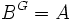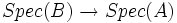# Automorphism group acts transitively on fibers of spectrum over fixed-point subring

(diff) ← Older revision | Latest revision (diff) | Newer revision → (diff)
This fact is an application of the following pivotal fact/result/idea: prime avoidance lemma
View other applications of prime avoidance lemma OR Read a survey article on applying prime avoidance lemma

## Statement

Let$G$ be a finite group acting as automorphisms of a commutative unital ring$B$, and let$A$ be the subring of$B$ comprising those elements that are fixed under every element of$G$ (i.e.$B^G = A$). Consider the map at the level of spectra:$Spec(B) \to Spec(A)$

There is a natural action of$G$ on the fibers over any point in$A$. The action is transitive.

## Proof

### Proof outline

• Consider the$G$-orbit of one element in the fiber
• Prove that any element in the fiber must, as a prime ideal, be contained in the union of all the prime ideals in that$G$-orbit. (This is the step where the main work is done)
• Apply the prime avoidance lemma